# Module SedimentQuality

## Overview

This is the 0D module that handles sediment biological processes made by bacteria as organic matter mineralization, nitrification, denitrification and immobilization.

## Main Processes

Main processes in SedimentQuality are organic matter mineralization, nitrification, denitrification and immobilization driven by microorganisms. These processes and microorganisms death are simulated using a standard rate (s.day-1.pop-1) that is afected by temperature, pH, oxygen in case of aerobic processes and substrate concentrations if exist. An old formulation based on RZWQM was adapted in order to be consistent in units and in the new formulation a maximum rate exists and "stress" factors (from 0 to 1) are computed accordingly to pH, aerobiose and aerobiose conditions, oxygen and susbtrate availability. Aerobiose and aerobiose conditions are computed from realtive water content.

A maximum rate is computed calculating the standard rate at the actual temperature (day-1.pop-1) times bacteria population that conducts the process.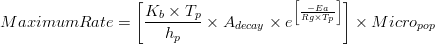$MaximumRate = \left [\frac{K_{b}\times T_{p}}{h_{p}}\times A_{decay}\times e^\left [{\frac{-Ea}{Rg\times T_{p}}} \right ] \right ]\times Micro_{pop}$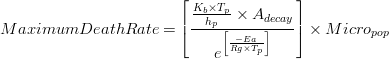$MaximumDeathRate = \left [\frac{\frac{K_{b}\times T_{p}}{h_{p}}\times A_{decay}}{e^\left [\frac{-Ea}{Rg\times T_{p}} \right ]} \right ]\times Micro_{pop}$


where:

Kb - Boltzman constant [J.K−1]
Tp - temperature [K]
hp - Planck constant [J.s]
Rg - Universal gas constant [Kcal.mole-1.K-1]
Adecay - decay coefficient [s. day-1.pop-1]
Ea - Apparent activation energy [Kcal.mole−1]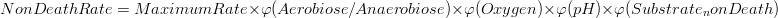$NonDeathRate = MaximumRate \times \varphi (Aerobiose/Anaerobiose)\times \varphi (Oxygen)\times \varphi (pH)\times \varphi (Substrate_nonDeath)$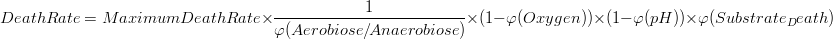$DeathRate = MaximumDeathRate \times \frac{1}{\varphi (Aerobiose/Anaerobiose)}\times (1 -\varphi (Oxygen))\times (1 -\varphi (pH))\times \varphi (Substrate_Death)$


pHTerm This term has a value of 1 in pHopt and symetric descending behaviour up to acid and basic pH's. In the case that is used in death rates the term is (1 - pHterm) since value 1 (maximum death rate) will be for the worst conditions (pH acid or basic) and 0 for best conditions.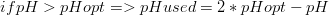$if pH > pHopt => pHused = 2* pHopt - pH$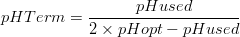$pHTerm=\frac{pHused}{2\times pHopt-pHused}$


OxygenTerm and Sustrate in non death rates The oxygen term and substrate term represent the abundance of substance that has not a negative effect on high concentrations. As so, for higher concentrations than optimum the value will be always 1. for lower concentrations than optimum there is a linear decrease of the term up to zero concentration and zero term. In the case that oxygen term is used in death rates it will become (1 - OxygenTerm) since the value 1 represents a maximum death rate and 0 represents no death.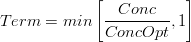$Term = min\left [\frac{Conc}{ConcOpt} , 1 \right ]$


SubstrateTerm in Death Rates In the case that the substrate term is used in death rates it becomes a exponential decay with increasing substrate (higher than a mimimum) to represent the same behaviour as old formulation. For concentration lower than the minimum, the term is 1 and death rate is maximum. For concentrations higher than the minimum the term as an exponential decay showing the effect of increasing material on lowering death rate.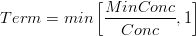$Term=min\left [\frac{MinConc}{Conc} , 1 \right ]$


### Organic Matter Mineralization

Organic matter is mineralized to ammonia with heterotrophic bacteria and also immobilization may occur in order to maintain bacteria C:N ratios.

LPOM mineralization rate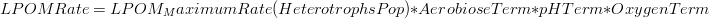$LPOMRate = LPOM_MaximumRate(HeterotrophsPop) * AerobioseTerm * pHTerm * OxygenTerm$


RPOM mineralization rate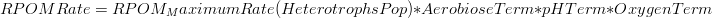$RPOMRate = RPOM_MaximumRate(HeterotrophsPop) * AerobioseTerm * pHTerm * OxygenTerm$


Immobilization rate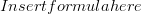$Insert formula here$


### Nitrification

Nitrification is driven by autotrophic microorganisms. Also immobilization is associated.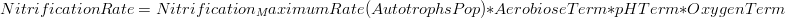$NitrificationRate = Nitrification_MaximumRate(AutotrophsPop) * AerobioseTerm * pHTerm * OxygenTerm$


### Denitrification

Denitrification is driven by anaerobic microorganisms. Also immobilization is associated.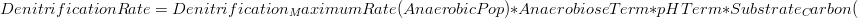$DenitrificationRate = Denitrification_MaximumRate(AnaerobicPop) * AnaerobioseTerm * pHTerm * Substrate_Carbon($


### Microorganisms Growth

Microorganisms incorporate carbon, nitrogen and phosphorus in the processes described above. However, they also have sinks as respiration and death.

Death Rates

Heterotrohpic microorganisms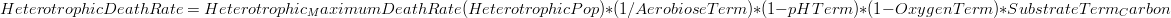$HeterotrophicDeathRate = Heterotrophic_MaximumDeathRate(HeterotrophicPop) * (1 /AerobioseTerm) * (1 - pHTerm) * (1 - OxygenTerm) * SubstrateTerm_Carbon$


Autotrohpic  microorganisms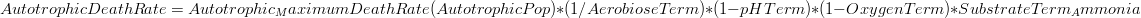$AutotrophicDeathRate = Autotrophic_MaximumDeathRate(AutotrophicPop) * (1 /AerobioseTerm) * (1 - pHTerm) * (1 - OxygenTerm) * SubstrateTerm_Ammonia$


Anaerobic  microorganisms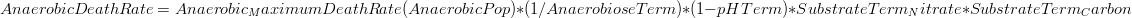$AnaerobicDeathRate = Anaerobic_MaximumDeathRate(AnaerobicPop) * (1 /AnaerobioseTerm) * (1 - pHTerm) * SubstrateTerm_Nitrate * SubstrateTerm_Carbon$


## Outputs

Output is done in terms of timeseries, HDF, and boxes following the MOHID standards.